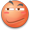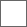### 出生年月日相同，我就去找你，我先来，2000+5+7=2011998+6+27＝20131420231998+2+16=2016

1999+8+8＝20152001+12+15=20281998+5+15=20151999+5+15=2019…所以话说楼上那个小姐姐我们好有缘。1999+11+9=2019

2000+5+6等于20561999+12+21=...2000+07+11=2018